# What are the 7 different types of angles?

• Difficulty Level : Easy
• Last Updated : 25 Mar, 2022

Geometry is the branch of mathematics, which deals with shapes and measurements like perimeter, area, volume related to them. When considering measurements the degree of inclination is very important of the figures as figures with different inclinations tend to differ in properties of area ad volume occupied by them. In the world of Mathematics, the inclination of two lines is given a formal name of an Angle. It denotes the degree of inclination of the two lines w.r.t each other. An angle is a measure formed when two rays meet at a common point (called Vertex).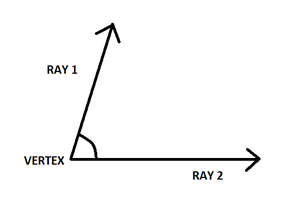### Angle

An angle according to euclidean geometry can be defined as the figure that is formed by two rays (by their intersection). The rays are known as the sides of the angle and the common point shared by the two rays is known as the vertex of the angle. Angle tells about the rotation and the amount of rotation of a certain parameter. Therefore, in physics, angular displacement and the terms used in the rotation part are concerned with angles. There are majorly three types of angles heard, they are right angle, acute angle, an obtuse angle. However, there are majorly seven types of angles present. Let’s learn about all the types of angles,

### What are the 7 different types of angles?

There are seven types of Angles commonly used in Mathematics:

1. Zero Angle (0° in Measure)
2. Acute Angle (0 to 90° in Measure)
3. Right Angle (90° in Measure)
4. Obtuse Angle (90 to 180° in Measure)
5. Straight Angle (180° in Measure)
6. Reflex Angle (180 to 360° in Measure)
7. Complete Angle (360° in Measure)

Below is an explanation of each of the different types of angles with their figures:

• Zero Angle: The two rays of the angle make zero degrees inclination w.r.t. each other i.e. the rays overlap. Here, Angle AOB denotes zero degrees in measure.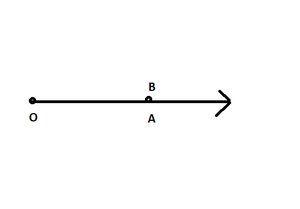Zero Angle

• Acute Angle: The two rays of the angle make zero to nighty degrees inclination. Here, Angle AOB denotes Acute degrees in measure.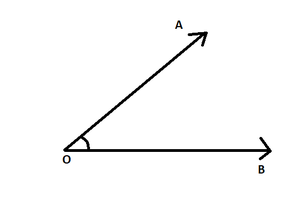Acute Angle

• Right Angle: The two rays of the angle make 90 degrees inclination. Here, Angle AOB denotes 90 degrees in measure.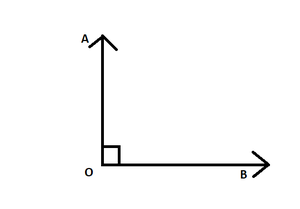Right Angle

• Obtuse Angle: The two rays of the angle make ninety to one-hundred eighty degrees inclination. Here, Angle AOB denotes Obtuse degrees in measure.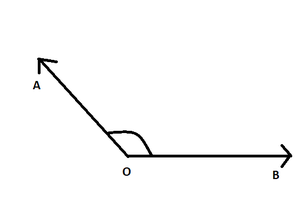Obtuse Angle

• Straight Angle: The two rays of the angle make one-hundred eighty degrees inclination or the rays are straight line w.r.t to each other. Here, Angle AOB denotes 180 degrees in measure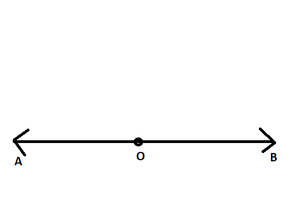Straight Angle

• Reflex Angle: The two rays of the angle make one-hundred-eighty to three hundred sixty degrees inclination. Here, Angle AOB denotes Reflex angle in measure.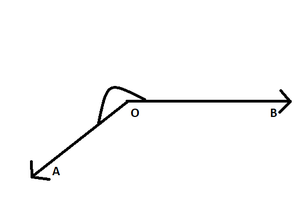Reflex Angle

• Complete Angle: The two rays of the angle make three hundred sixty degrees inclination. Here, Angle AOB denotes Complete angle in measure.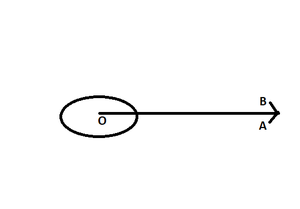Complete Angle

### Sample Problems

Question 1: An Angle A measures 135° in measure. Which type of category does Angle A fall into?

Angle A lies between 90° to 180° in measure. Angle A is, therefore, an Obtuse Angle.

Question 2: An Angle S measures 35° in measure. Which type of category Angle S falls into?

Angle S lies between 0° to 90° in measure. Angle S is therefore an Acute Angle.

Question 3: An Angle X measures 235 degrees in measure. Which type of category Angle X falls into?